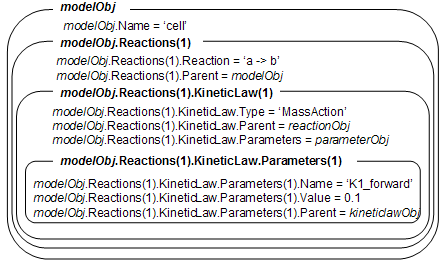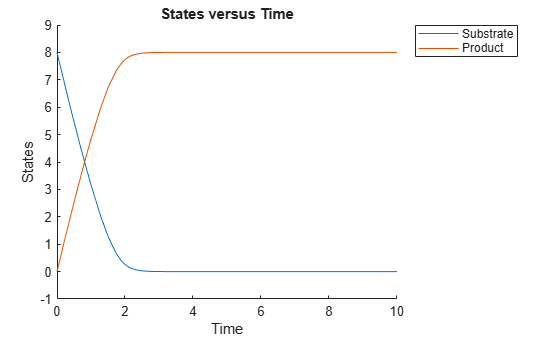Create kinetic law object and add to reaction object

## Syntax

```kineticlawObj = addkineticlaw(reactionObj, 'KineticLawNameValue') kineticlawObj= addkineticlaw(..., 'PropertyName', PropertyValue, ...) ```

## Arguments

 `reactionObj` Reaction object. Enter a variable name for a reaction object. `KineticLawNameValue` Property to select the type of kinetic law object to create. For built-in kinetic law, valid values are: `'Unknown'`, `'MassAction'`, `'Henri-Michaelis-Menten'`, `'Henri-Michaelis-Menten-Reversible'`, `'Hill-Kinetics`', `'Iso-Uni-Uni'`, `'Ordered-Bi-Bi'`, `'Ping-Pong-Bi-Bi'`, `'Competitive-Inhibition'`, `'NonCompetitive-Inhibition'`, and `'UnCompetitive-Inhibition'`. Find valid `KineticLawNameValue` by using `sbioroot` to create a SimBiology® root object, then query the object with the commands `rootObj.BuiltinLibrary.KineticLaws` and `rootObj.UserDefinedLibrary.KineticLaws`.```sbiowhos -kineticlaw``` lists kinetic laws in the SimBiology root, which includes kinetic laws from both the `BuiltInLibrary` and the `UserDefinedLibrary`.

## Description

```kineticlawObj = addkineticlaw(reactionObj, 'KineticLawNameValue')``` creates and adds a `KineticLaw object` to the `reactionObj`.

In the kinetic law object, this method assigns a name (`KineticLawNameValue`) to the property `KineticLawName` and assigns the reaction object to the property `Parent`. In the reaction object, this method assigns the kinetic law object to the property `KineticLaw`.

```modelObj = sbiomodel('cell'); reactionObj = addreaction(modelObj, 'a -> b'); kineticlawObj = addkineticlaw(reactionObj, 'MassAction'); parameterObj = addparameter(kineticlawObj, 'K1_forward', 0.1); set(kineticlawObj, ParameterVariableName, 'K1_forward'); ````KineticLawNameValue` is any valid kinetic law definition. See Kinetic Law Definition for a definition of kinetic laws and more information about how they are used to get the reaction rate expression.

``` kineticlawObj= addkineticlaw(..., 'PropertyName', PropertyValue, ...)``` constructs a kinetic law object, `kineticlawObj`, and configures `kineticlawObj` with property value pairs. The property name/property value pairs can be in any format supported by the function `set`. The `kineticlawObj` properties are listed in Property Summary.

### Note

To define a Hill kinetic rate equation with a non-integer exponent that is compatible with `DimensionalAnalysis`, see Define a Custom Hill Kinetic Law that Works with Dimensional Analysis.

## Property Summary

Properties for kinetic law objects

 Expression (AbstractKineticLaw, KineticLaw) Expression to determine reaction rate equation KineticLawName Name of kinetic law applied to reaction Name Specify name of object Notes HTML text describing SimBiology object ParameterVariableNames Cell array of reaction rate parameters ParameterVariables Parameters in kinetic law definition Parameters Array of parameter objects Parent Indicate parent object SpeciesVariableNames Cell array of species in reaction rate equation SpeciesVariables Species in abstract kinetic law Tag Specify label for SimBiology object Type Display SimBiology object type UserData Specify data to associate with object

## Examples

collapse all

This example shows how to simulate the conversion of a substrate into a product using the Henri-Michaelis-Menten enzyme kinetics.

Create a model named `mymodel`.

`model = sbiomodel('mymodel');`

Add a reaction that represents the conversion of a substrate to a product.

`reaction = addreaction(model,'Substrate -> Product');`

Add the built-in Henri-Michaelis-Menten kinetic law to the reaction.

```kineticLaw = addkineticlaw(reaction,'Henri-Michaelis-Menten'); kineticLaw.Expression```
```ans = 'Vm*S/(Km + S)' ```

The kinetic law has two parameters and a species that you need to define. View these parameters.

`kineticLaw.ParameterVariables`
```ans = 2x1 cell {'Vm'} {'Km'} ```
`kineticLaw.SpeciesVariables`
```ans = 1x1 cell array {'S'} ```

To define the parameters, create two parameter objects and set parameter values.

```Vm_param = addparameter(kineticLaw,'Vm_param','Value',6.0); Km_param = addparameter(kineticLaw,'Km_param','Value',1.25);```

Map the parameters accordingly by setting the `ParameterVariableNames` property. This associates the parameters in the expression with the two parameters you just created using a one-to-one mapping in the order given.

`kineticLaw.ParameterVariableNames = {'Vm_param','Km_param'};`

Also associate the `Substrate` species with the species `S` in the expression.

`kineticLaw.SpeciesVariableNames = {'Substrate'};`

Verify the mapping by looking at the reaction rate and checking the parameters and species are correctly substituted according to the expression.

`reaction.ReactionRate`
```ans = 'Vm_param*Substrate/(Km_param+Substrate)' ```

Enter the initial amount of the substrate species for simulation.

`model.Species(1).InitialAmount = 8;`

Simulate the model and plot results.

```simdata = sbiosimulate(model); sbioplot(simdata);```